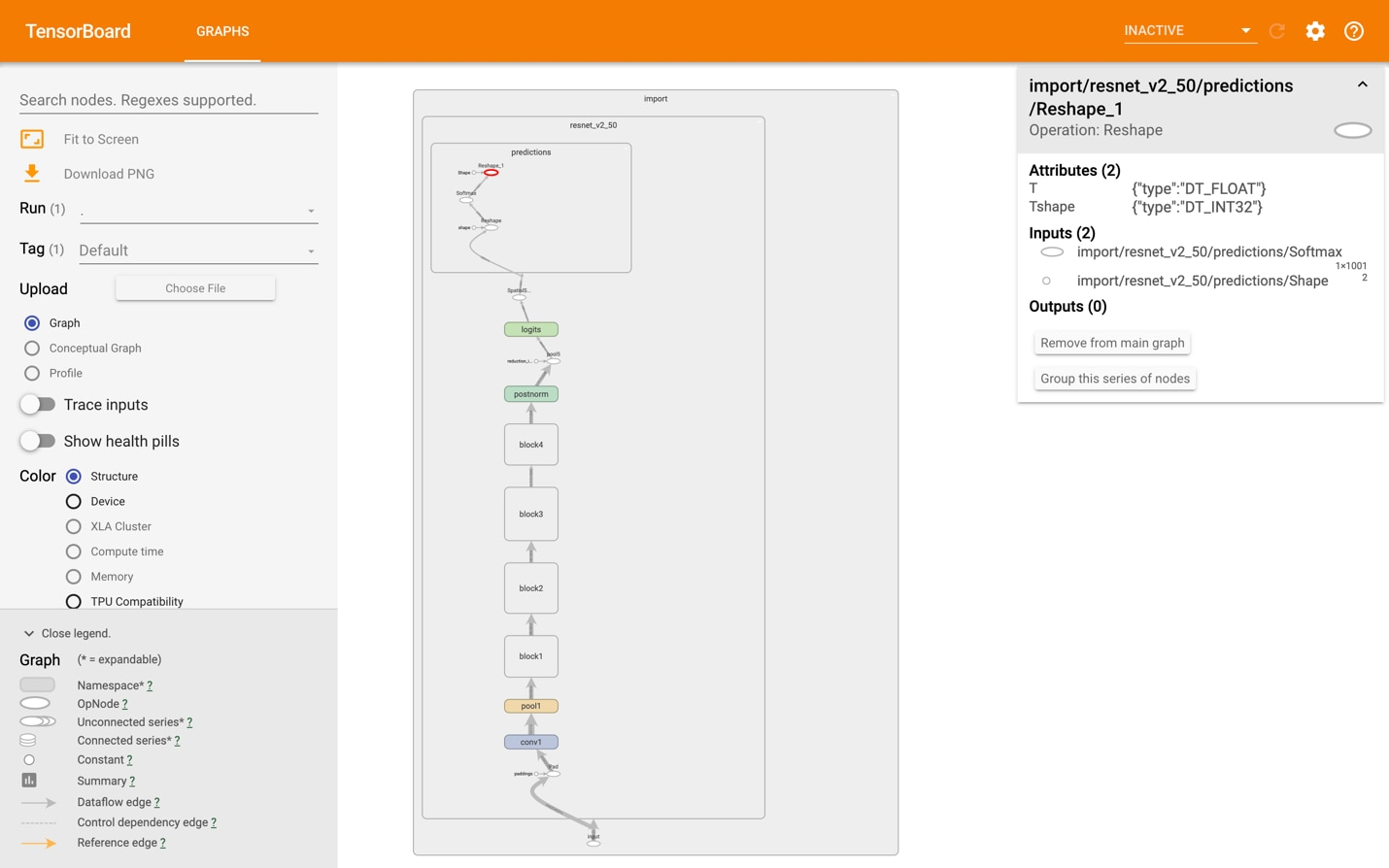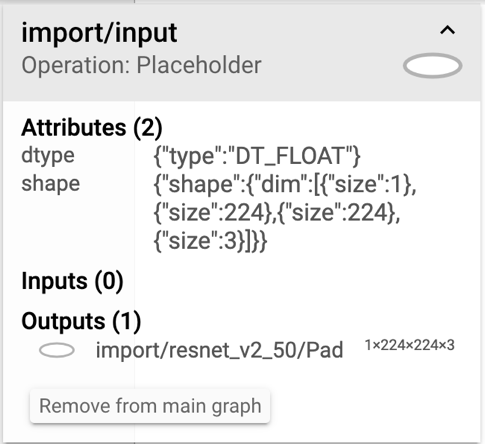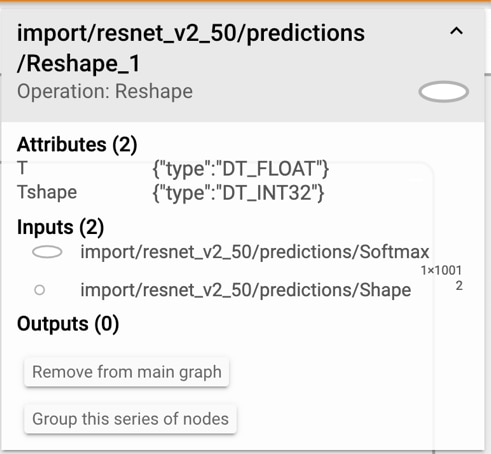## Determine the names of input and output nodes

Skip this step if you can determine the names of the input and output nodes from the provider of your model or the training code. However, this step also demonstrates how to visualize the computational graph in a neural network model. This will help you to understand what will be executed at runtime and how the various transform_graph operations affect the structure of the model in practice.

The simplest way to visualize graphs is to use TensorBoard. To install TensorBoard, enter the following on the command line:

`pip3 install tensorboard`
`tensorboard --logdir=/tmp/tensorboard`

Download resnet_v2_50.pb from here. Use the script provided in the TensorFlow source distribution to import model (.pb) files to TensorBoard by entering the following on the command line:

`python3 tensorflow_core/python/tools/import_pb_to_tensorboard.py --model_dir resnet_v2_50.pb --log_dir /tmp/tensorboard`
`tensorboard --logdir=/tmp/tensorboard`

Do not let the name of the argument model_dir confuse you. A .pb file is an acceptable target.

Important: If you repeat this command for importing multiple models, empty the /tmp/tensorboard directory after each import to prevent confusion.

In a browser, navigate to http://localhost:6006/ and select the GRAPHS tab to see the model's graph. If it is collapsed under a single node, as shown in this image, use the expand control until you get to the actual operations.In this network, we can see that Placeholder is the only input node and Reshape_1 is the output node. To get their full names, select them and look at the details box on the right, as this image shows.Here, the name of the input node is import/input/Placeholder and the output node is import/resnet_v2_50/predictions/Reshape_1.

The details box also shows that this model has been trained with the standard 224x224x3 input size which is typical for a ResNet architecture. The transform_graph tool assumes the import namespace, so these are simplified to:

• Input name: Placeholder.
• Input dimensions: 1x224x224x3.
• Output name: resnet_v2_50/predictions/Reshape_1.

We can also use the summarize_graph tool to inspect the model and provide guesses about likely input and output nodes, as well as other information that is useful for debugging. Here is an example of how to use it on the resnet_v2_50 graph:

`\$ bazel build tensorflow/tools/graph_transforms:summarize_graph`
`\$ bazel-bin/tensorflow/tools/graph_transforms/summarize_graph --in_graph=resnet_v2_50.pb`
`Found 1 possible inputs: (name=input, type=float(1), shape=[1,224,224,3]) `
`No variables spotted.`
`Found 1 possible outputs: (name=resnet_v2_50/predictions/Reshape_1, op=Reshape) `
`Found 25615936 (25.61M) const parameters, 0 (0) variable parameters, and 0 control_edges`
`Op types used: 328 Const, 272 Identity, 147 Mul, 114 Add, 54 Conv2D, 49 Relu, 49 Rsqrt, 49 Sub, 22 BiasAdd, 4 MaxPool, 4 Pad, 2 Reshape, 1 Mean, 1 Placeholder, 1 Softmax, 1 Squeeze`

To use with

`tensorflow/tools/benchmark:benchmark_model`

try these arguments:

`bazel run tensorflow/tools/benchmark:benchmark_model -- --graph=resnet_v2_50.pb --show_flops --input_layer=input --input_layer_type=float --input_layer_shape=1,224,224,3 --output_layer=resnet_v2_50/predictions/Reshape_1`